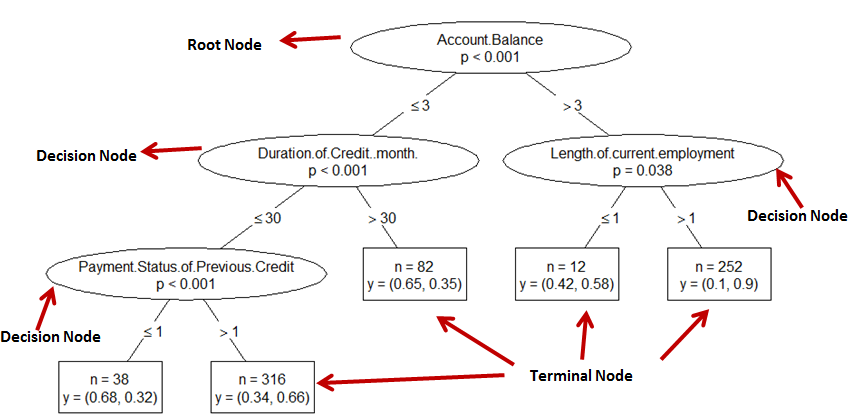# How to Customize CTREE Decision Tree Plot in R

Suppose you want to change a look of default decision tree generated by CTREE function in the party package in R.Decision Tree Custom Ctree Plot
Create Sample Data

Let's create a sample data for demonstration purpose. Here we created a binary variable for using it as a target variable in the decision tree.

```library(dplyr)
iris2 <- iris %>%
mutate(Target = as.factor(ifelse(Species == "setosa", "Setosa", "Not Setosa"))) %>%
select(-Species)
```

The ctree function is used to create the tree and then the plot function is used with custom panel functions to customize the look of the tree.

```library(party)
t1.prior <- ctree(Target ~ ., data = iris2)
plot(t1.prior, type="simple",
inner_panel=node_inner(t1.prior,abbreviate = FALSE, pval = TRUE, id = FALSE),
terminal_panel=node_terminal(t1.prior, abbreviate = FALSE, digits = 2, fill = c("white"),id = FALSE)
)
```
How does the above code work?
1. `ctree(Target ~ ., data = iris2):` This line creates a conditional inference tree model with the binary target variable `Target` and all other variables in the `iris2` dataset as independent variables.
2. `plot(...):` This is used to plot the created tree with the following customizations.
• `type="simple":` Specifies the type of plot, which is a simple plot.
• `inner_panel=...:` Specifies the custom panel for inner nodes using the `node_inner` function. The `abbreviate` argument is set to `FALSE` to display full variable names, `pval = TRUE` displays p-values, and `id = FALSE` hides the node IDs.
• `terminal_panel=...:` Specifies the custom panel for terminal nodes using the `node_terminal` function. The `abbreviate` argument is set to `FALSE` to display full variable names, `digits = 2` sets the number of digits displayed, `fill = c("white")` sets the fill color for terminal nodes, and `id = FALSE` hides the node IDs.
Related Posts